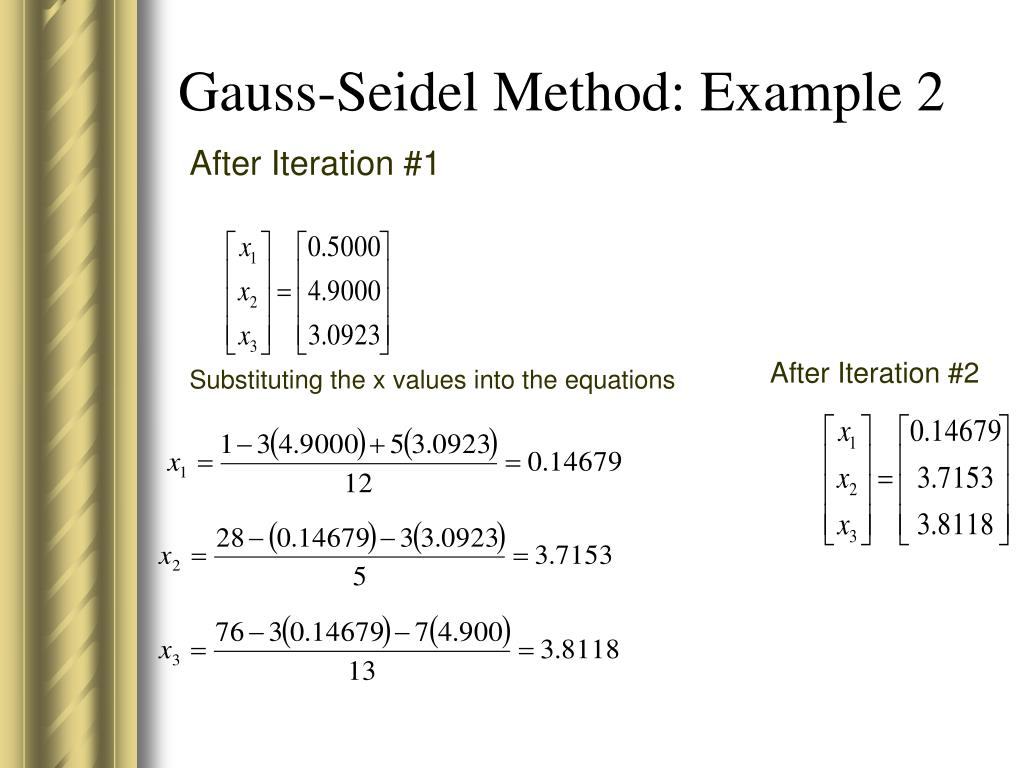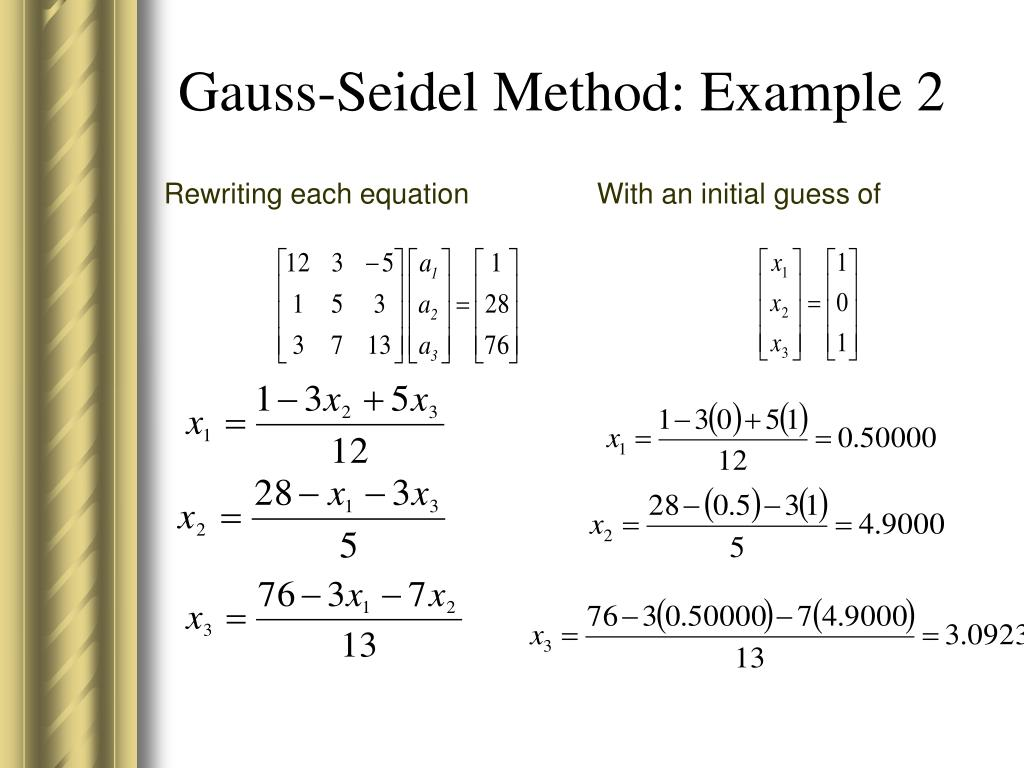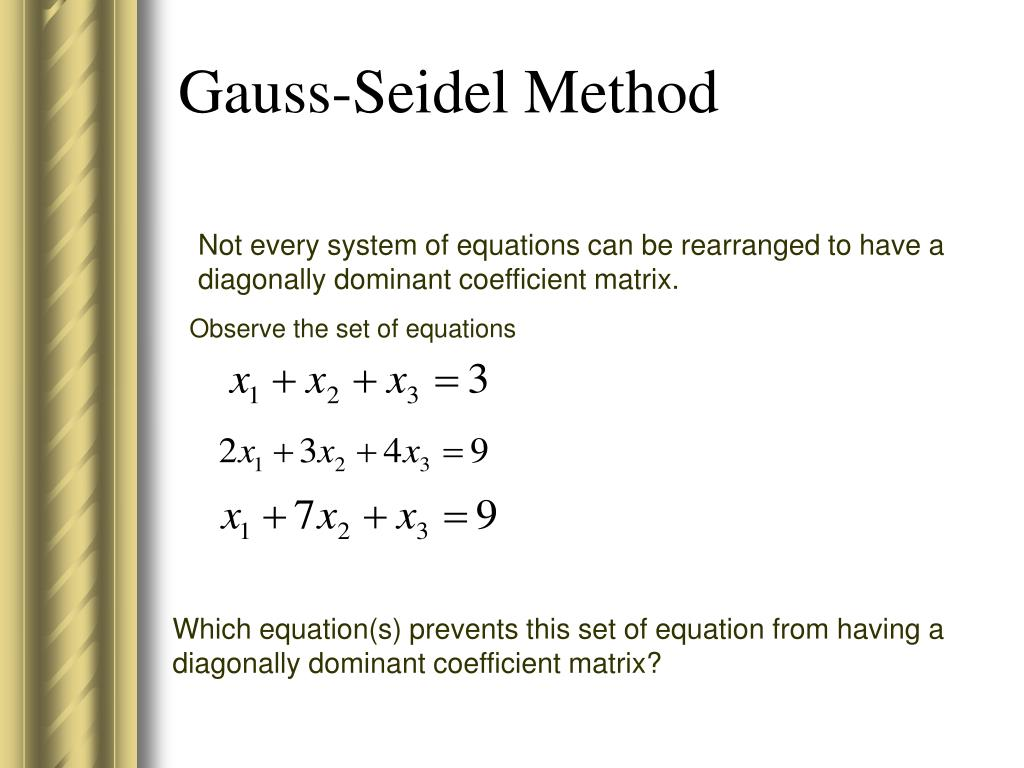# Gauss Seidal Method Presentation

Introduction to Gauss Seidel Method
The Gauss Seidel Method is an iterative numerical method used to solve systems of linear equations.

It is an improvement over the Gauss elimination method, as it converges faster for certain types of equations.

The method is named after the German mathematicians Carl Friedrich Gauss and Philipp Ludwig von Seidel.
Image Source1

Algorithm of the Gauss Seidel Method

For each equation in the system, update the solution vector using the values from the previous iteration.

Repeat the process until the desired level of convergence is achieved or a maximum number of iterations is reached.
Image Source2

Faster convergence: The Gauss Seidel Method often converges faster for diagonally dominant or symmetric positive definite matrices.

Memory efficient: Unlike the Gauss elimination method, the Gauss Seidel Method does not require storing the entire matrix.

Suitable for parallel computing: The method can be easily parallelized, allowing for efficient use of multiple processors or threads.
Image Source3

Limitations of Gauss Seidel Method
Slower convergence for non-diagonally dominant matrices: In some cases where the matrix is not diagonally dominant, the method may converge slowly or even fail to converge.

Requires knowledge of equation coefficients: The method requires knowing the coefficients of the equations in advance.

Not suitable for large-scale problems: For very large systems of equations, the Gauss Seidel Method may not be the most efficient choice due to its iterative nature.
Image Source4

Applications of Gauss Seidel Method
Circuit analysis: The method can be used to solve electrical circuit systems with resistors, capacitors, and inductors.

Finite Element Analysis: The Gauss Seidel Method is used in solving partial differential equations in the field of structural analysis and heat transfer.

Image processing: The method finds application in image denoising, image reconstruction, and image segmentation algorithms.
Image Source5